# Class 10 Maths Worksheet for Similar triangles

Question 1.
ABC is an isosceles triangle, right -angled at C. Prove that $AB^2 = 2BC^2$.
Solution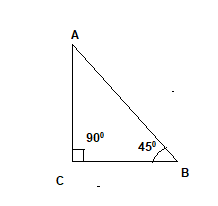Now Since it is an isosceles triangle
AC=BC -(1)
Now from Pythagorean theorem
$AB^2 = AC^2 + BC^2$
From
$AB^2 = 2BC^2$

Question 2.
The areas of two similar triangles ABC and PQR are in the ratio 9 : 16. If BC = 4.5 cm, find the length of QR.
Solution
We know that
$\frac {ar( \Delta ABC)}{ar( \Delta PQR)}=\frac {BC^2}{QR^2}$
$\frac {9}{16} = \frac {4.5^2}{QR^2}$
$QR= 6 cm$

Question 3.
The foot of a ladder is 6 m away from a wall and its top reaches a window 8 m above the ground. If the ladder is shifted in such a way that its foot is 8 m away from the wall, to what height does its tip reach?
Solution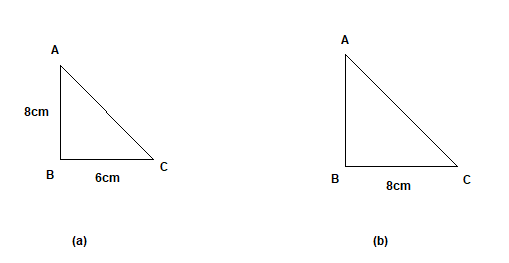First case is depicted in the figure (a)
Now from Pythagoras theorem
$AC^2 = AB^2 + BC^2$
$AC^2 = 8^2 + 6^2 =100$
$AC=10$ cm
This is the length of the ladder.

Now Second case is depicted in figure (b)
AC=10 cm
BC=8 cm
Now from Pythagoras theorem
$AC^2 = AB^2 + BC^2$
$AB^2 = AC^2 - BC^2=10^2 - 8^2 =36$
$AB=6$ cm

Question 4.
Determine whether the triangle having sides $(b - 1)$ cm, $2\sqrt {b}$ cm and $(b + 1)$ cm is a right angled triangle.
Solution
These are sides of the Right angle triangle
$(b+1)^2 = (2\sqrt {b})^2 + (b-1)^2$
$(b+1)^2 = 4b + b^2 +1 -2b$
$(b+1)^2=b^2 + 1 +2 b$
$(b+1)^2 = (b+1)^2$

Question 5.
The areas of two similar triangles are 121 cm2 and 64 cm2 respectively. If the median of the first triangle is 12.1 cm, find the corresponding median of the other.
Solution
We know that
$\frac {ar( \Delta 1)}{ar( \Delta 2)}=\frac {M_1^2}{M_2^2}$
$\frac {121}{64} = \frac {12.1^2}{QR}$
$QR= 8.8 cm$

Question 6.
In $\Delta ABC$, AD is perpendicular to BC. Prove that:
a. $AB^2+ CD^2 = AC^2 + BD^2$
b. $AB^2 - BD^2 = AC^2 - CD^2$
Solution

In $\Delta ABC$, AD is perpendicular to BC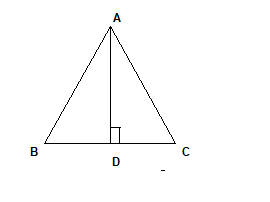Now $\Delta ABD$ is an right -angle triangle
So from Pythagoras theorem
$AB^2 = AD^2 + BD^2$
$AD^2 = AB^2 - BD^2$ -(1)
Similarly $\Delta ADC$ is an right -angle triangle
So from Pythagoras theorem
$AC^2 = AD^2 + CD^2$
$AD^2 = AC^2 - CD^2$ --(2)
From (1) and (2)
$AB^2 - BD^2 = AC^2 - CD^2$
Which proved part (b)
Now rearranging,
$AB^2+ CD^2 = AC^2 + BD^2$
Which proved part (a)

Question 7.
In a quadrilateral ABCD, given that $\angle A + \angle D = 90^0$. Prove that $AC^2 + BD^2 = AD^2 + BC^2$
Solution
ABCD is a quadrilateral as shown below. Extending the side CD and AB to meet at point P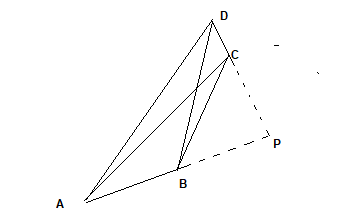Now in $\Delta APD$
$\angle A + \angle D + \angle C =180$
Now $\angle A + \angle D = 90^0$
Therefore
$\angle C=90$

Now in right angle triangle APC
$AC^2 = AP^2 + PC^2$
Now in right angle triangle BPD
$BD^2 = BP^2 + DP^2$
$AC^2 + BD^2 =AP^2 + PC^2 + BP^2 + DP^2$ -(1)
Now in right angle triangle APD
$AD^2 = AP^2 + DP^2$
Now in right angle triangle BCP
$BC^2 = CP^2 + BP^2$
$AD^2 + BC^2 = AP^2 + PC^2 + BP^2 + DP^2$ -(2)
From (1) and (2)
$AC^2 + BD^2 = AD^2 + BC^2$

Question 8.
Prove that a line drawn through the mid- point of one side of a triangle parallel to another side bisects the third side.
Question 9.
Prove that the line joining the mid – points of any two sides of a triangle is parallel to the third side

Question 10.
Sides of triangles are given below. Determine which of them are right triangles.In case of a right triangle, write the length of its hypotenuse.
(i) 7 cm, 24 cm, 25 cm
(ii) 3 cm,4 cm,5 cm
(iii) 40 cm, 80 cm, 100 cm
(iv) 13 cm, 12 cm, 5 cm
Solution
i. $25^2 = 7^2 + 24^2$
Hence right angle triangle with 25 cm as hypotenuse
ii. $5^2 = 3^2 + 4^2$
Hence right angle triangle with 5 cm as hypotenuse
iii. $100^2 = 40^2 + 80^2$
Hence right angle triangle with 100 cm as hypotenuse
iv. $13^2 = 12^2 + 5^2$
Hence right angle triangle with 13 cm as hypotenuse
Question 11.
DEF is an equilateral triangle of side $2b$. Find each of its altitudes.
Solution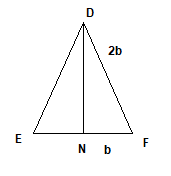In right angle triangle DNF
$DF^2 = DN^2 + NF^2$
$DN^2 = DF^2 - NF^2$
$DN^2 = 4b^2 - b^2$
$DN =b \sqrt {3}$

Question 12.
Triangle ABC is right- angled at B and D is the mid - point of BC.
Prove that: $AC^2 = 4AD^2 - 3AB^2$
Solution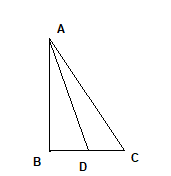In right angle triangle ABC
$AC^2 = AB^2 + BC^2$ --(1)
In right triangle ABD
$AD^2 = AB^2 + BD^2$
$AD^2 =AB^2 + \frac {BC^2}{4}$
$BC^2 = 4AD^2 - 4 AB^2$
Substituting this in equation (1)
$AC^2 = 4AD^2 - 3AB^2$

Question 13.
The sides of a triangle ABC are in the ratio AB : BC : CA = 1 : √2: 1. Show that ABC is a right triangle, right -angled at A.
Solution
AB : BC : CA = 1 : √2: 1
$AB=x$
$BC= x\sqrt {2}$
$CA=x$
Now
$BC^2 = AB^2 + AC^2$
$2x^2 = x^2 +x^2$
$2x^2=2x^2$
So ABC is right -angled at A

Question 14.
A vertical pole of length 6 m casts a shadow 4 m long on the ground and at the same time a tower casts a shadow 28 m long. Find the height of the tower
Solution
Given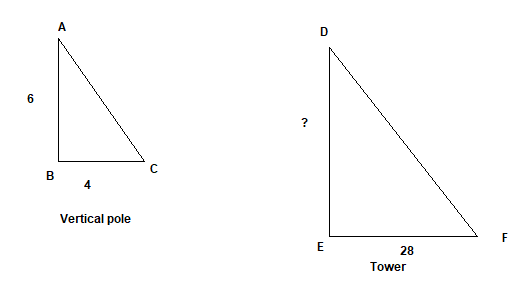AB = 6m
BC= 4 m
Let Height of tower (DE)=h m
EF = 28 m
In $\Delta ABC$ and $\Delta DEF$
$\angle C = \angle F$ (Same angle of elevation of Sun)
$\angle B = \angle E = 90$
By AA similarity criterion
$\Delta ABC \sim \Delta DEF$

$\frac {AB}{DE}=\frac {BC}{EF}$
$h=42 m$

Go back to Class 10 Main Page using below links

### Practice Question

Question 1 What is $1 - \sqrt {3}$ ?
A) Non terminating repeating
B) Non terminating non repeating
C) Terminating
D) None of the above
Question 2 The volume of the largest right circular cone that can be cut out from a cube of edge 4.2 cm is?
A) 19.4 cm3
B) 12 cm3
C) 78.6 cm3
D) 58.2 cm3
Question 3 The sum of the first three terms of an AP is 33. If the product of the first and the third term exceeds the second term by 29, the AP is ?
A) 2 ,21,11
B) 1,10,19
C) -1 ,8,17
D) 2 ,11,20

Latest Articles
Synthetic Fibres and Plastics Class 8 Practice questions

Class 8 science chapter 5 extra questions and Answers

Mass Calculator

3 Fraction calculator

Garbage in Garbage out Extra Questions7